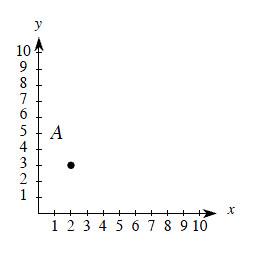### Home > MC2 > Chapter 2 > Lesson 2.1.2 > Problem2-32

2-32.

One of the topics you will soon review in Section 2.2 is graphing shapes. The graph at right is called a first quadrant graph. It uses two number lines that only show positive numbers and zero. .

1. Carefully copy the axes (the number lines) and point A on a piece of graph paper.

2. Plot the points listed below. Then connect them in the order given. Point A is given for you.
$A$ $\left(2, 3\right)$, $B$ $\left(3, 7\right)$, $C$ $\left(7, 7\right)$, $D$ $\left(8, 3\right)$ Then connect $D$ to $A$.

3. What is the name of the shape that you drew?

Which quadrilateral has only one pair of parallel sides?Use the eTool below to plot the points.
Click the link at right for the full version of the eTool: MC2 2-32 HW eTool